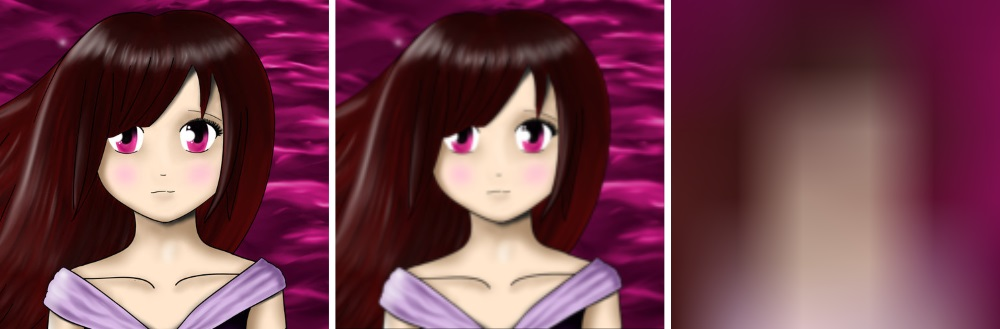OpenCV  3.4.7 Open Source Computer Vision
Filtering using F-transform

# Goal

This tutorial demonstrates to you how to use F-transform for image filtering. You will see:

• basic theory behind,
• illustration of different settings.

# Fuzzy transform application

As I shown in previous tutorial, F-transform is a tool of fuzzy mathematics highly usable in image processing. Let me rewrite the formula using kernel $$g$$ introduced before as well:

$F^0_{kl}=\frac{\sum_{x=0}^{2h+1}\sum_{y=0}^{2h+1} \iota_{kl}(x,y) g(x,y)}{\sum_{x=0}^{2h+1}\sum_{y=0}^{2h+1} g(x,y)},$

where $$\iota_{kl} \subset I$$ centered to pixel $$(k \cdot h,l \cdot h)$$ and $$g$$ is a kernel. More details can be found in related papers.

# Code

/* Sample - Filtering
* Target is to apply filtering using F-transform
* on the image "input.png". Two different kernels
* are used, where bigger radius (100 in this case)
* means higher level of blurriness.
*
* Image "output1_filter.png" is created from "input.png"
* using "kernel1" with radius 3.
*
* Image "output2_filter.png" is created from "input.png"
* using "kernel2" with radius 100.
*
* Both kernels are created from linear function, using
* linear interpolation (parameter ft:LINEAR).
*/
#include "opencv2/core.hpp"
using namespace std;
using namespace cv;
int main(void)
{
// Input image
// Kernel creation
Mat kernel1, kernel2;
ft::createKernel(ft::LINEAR, 3, kernel1, 3);
ft::createKernel(ft::LINEAR, 100, kernel2, 3);
// Filtering
Mat output1, output2;
ft::filter(I, kernel1, output1);
ft::filter(I, kernel2, output2);
// Save output
imwrite("output1_filter.png", output1);
imwrite("output2_filter.png", output2);
return 0;
}

# Explanation

Image filtering changes input in a defined way to enhance or simply change some concrete feature. Let me demonstrate some simple blur.

As a first step, we load input image.

// Input image

Following the F-transform formula, we must specify a kernel.

// Kernel cretion
Mat kernel1, kernel2;
ft::createKernel(ft::LINEAR, 3, kernel1, 3);
ft::createKernel(ft::LINEAR, 100, kernel2, 3);

So now, we have two kernels that differ in radius. Bigger radius leads to bigger blur.

The filtering itself is applied as shown below.

// Filtering
Mat output1, output2;
ft::filter(I, kernel1, output1);
ft::filter(I, kernel2, output2);

Output images look as follows.